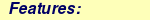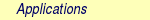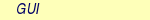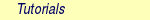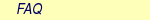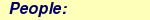MOLCAS manual:Next: 8.46 SlapAf Up: 8. Programs Previous: 8.44 seward

Subsections

# 8.45 single_aniso

The SINGLE_ANISO program is a routine which allows the non-perturbative calculation of effective spin (pseudospin) Hamiltonians and static magnetic properties of mononuclear complexes and fragments completely ab initio, including the spin-orbit interaction. As a starting point it uses the results of RASSI calculation for the ground and several excited spin-orbital multiplets. A short description of methodology and applications can be found in , . The second version of the SINGLE_ANISO program is able to calculate the following quantities:

• Parameters of pseudospin magnetic Hamiltonians (the methodology is described in ):
1. First rank (linear after pseudospin) Zeeman splitting tensor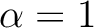, its main values, including the sign of the product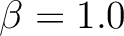, and the main magnetic axes.
2. Second rank (bilinear after pseudospin) zero-field splitting tensor, its main values and the anisotropy axes. The anisotropy axes are given in two coordinate systems: a) in the initial Cartesian coordinate system (x, y, z) and b) in the coordinate system of the main magnetic axes (Xm, Ym, Zm).
3. Higher rank ZFS tensors (D4, D6, ... , etc.) and Zeeman splitting tensors (G3, G5, ... , etc.) for complexes with moderate and strong spin-orbit coupling.
4. Angular moments along the main magnetic axes.

• All (27) parameters of the ab initio Crystal field acting on the ground atomic multiplet of lanthanides, and the decomposition of the CASSCF/RASSI wave functions into functions with definite projections of the total angular moment on the quantization axis.

• Static magnetic properties:
1. Van Vleck susceptibility tensor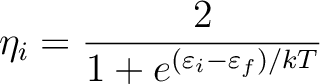2. Powder magnetic susceptibility function3. Magnetization vector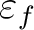for specified directions of the applied magnetic field4. Powder magnetization Mmol(H)

The magnetic Hamiltonians are defined for a desired group of N electronic states obtained in RASSI calculation to which a pseudospin S (it reduces to a true spin S in the absence of spin-orbit coupling) is subscribed according to the relation N=2S+1. For instance, the two wave functions of a Kramers doublet correspond to S=1/2. The implementation is done for S=1/2, 1, 3/2, ... ,15/2.

The calculation of magnetic properties takes into account the contribution of excited states (the ligand-field and charge transfer states of the complex or mononuclear fragment included in the RASSI calculation) via their thermal population and Zeeman admixture. The intermolecular exchange interaction between magnetic molecules in a crystal can be taken into account during the simulation of magnetic properties by a phenomenological parameter zJ specified by the user (see keyword MLTP).

## 8.45.1 Dependencies

The SINGLE_ANISO program takes all needed ab initio information from the RUNFILE: i.e. matrix elements of angular momentum, spin-orbit energy spectrum and mixing coefficients, number of mixed states and their multiplicity, etc. In order to find the necessary information in the RUNFILE, the keywords MEES and SPIN are mandatory for RASSI. The SEWARD keyword ANGM is also compulsory.

## 8.45.2 Files

### 8.45.2.1 Input files

 File Contents RUNFILE The file of communication between different modules in MOLCAS. Its presence is mandatory.

### 8.45.2.2 Output files

 File Contents \$PROJECT.ANISO This file is intended to be an input for the future POLY_ANISO module in MOLCAS (in development). The creation of this file is done via a specific keyword.

## 8.45.3 Input

Normally SINGLE_ANISO runs without specifying any of the following keywords. The only unknown variable for SINGLE_ANISO is the dimension (multiplicity) of the pseudospin. By default one multiplet is selected, which has the dimension equal to the multiplicity of the ground term. For example, in cases where spin-orbit coupling is weak, the multiplicity of the effective spin Hamiltonian is usually the same as the multiplicity of the lowest term, while in the cases with strong anisotropy (lanthanide or actinide complexes, Co2+ complexes, etc...) the lowest energy levels of the complexes form a group of states which can differ quite strong from the spin multiplicity of the lowest term. In these cases the user should specify the multiplicity corresponding to a chosen value of pseudospin (2S+1). For instance, in Dy3+ the spin of the ground state term is S=5/2, but in many situations only the ground Kramers doublet is considered; then the user should set the multiplicity of the pseudospin equal to 2 (see MLTP keyword). The calculation of the parameters of the crystal field corresponding to the ground atomic multiplet for lanthanides should be requested by the CRYS keyword.

&SINGLE_ANISO

Argument(s) to a keyword are always supplied on the next line of the input file.

### 8.45.3.1 Optional general keywords to control the input

 Keyword Meaning TITLe One line following this one is regarded as title. TYPE Specifies which magnetic properties must be computed. The program will read one of the following numbers (1-7): 1: - the g- and D-tensors (only) 2: - the powder magnetic susceptibility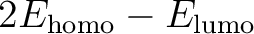, the magnetic susceptibility tensor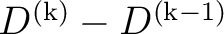, magnetic susceptibility in the direction of the main magnetic axis:,,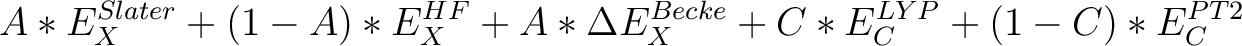3: - the powder molar magnetization M(H), the magnetization vectors MX(H), MY(H), MZ(H) -for certain directions of the field Hi. 4: - 1 + 2 5: - 1 + 3 6: - 2 + 3 7: - 1 + 2 + 3. This is the default value. MLTP The number of molecular multiplets (i.e. groups of spin-orbital eigenstates) for which g, D and higher magnetic tensors will be calculated (default MLTP=1). The program reads two lines: the first is the number of multiplets (NMULT) and the second the array of NMULT numbers specifying the dimension of each multiplet. The default is to select one multiplet which has the dimension equal to the multiplicity of the ground term. In cases of strong spin-orbit coupling the usage of this keyword is mandatory. Example: MLTP 4 4  4  2  2 SINGLE_ANISO will compute the g tensor for 4 groups of states: 2 groups having the effective spin S=|3/2>, and other 2 groups of states being Kramers doublets. TINT Specifies the temperature points for the evaluation of the magnetic susceptibility. The program will read four numbers: Tmin, Tmax, nT, and. Tmin - the minimal temperature (Default 0.0K) Tmax - the maximal temperature (Default 300.0K) nT - number of temperature points (Default 101)- the minimal temperature above the 0.0K that helps to avoid infinite values during calculation (Default 0.001K). Example: TINT 0.0  330.0  331  0.0001 SINGLE_ANISO will compute temperature dependence of the magnetic susceptibility in 331 points evenly distributed in temperature interval: 0.0K - 330.0K.=0.0001K. HINT Specifies the field points for the evaluation of the magnetization in a certain direction. The program will read four numbers: Hmin, Hmax, nH, and. Hmin - the minimal field (Default 0.0T) Hmax - the maximal filed (Default 10.0T) nH - number of field points (Default 101)- the minimal field above the 0.0T that helps to avoid infinite values during calculation (Default 0.001T). Example: HINT 0.0  20.0  201  0.0001 SINGLE_ANISO will compute the molar magnetization in 201 points evenly distributed in field interval: 0.0T - 20.0T.=0.0001T. TMAG Specifies the temperature at which the field-dependent magnetization is calculated. Default is 2.0 K Example: TMAG 1.8 ENCU This flag is used to define the cut-off energy for the lowest states for which Zeeman interaction is taken into account exactly. The contribution to the magnetization coming from states that are higher in energy than E (see below) is done by second order perturbation theory. The program will read two integer numbers: NK and MG. Default values are: NK=100,MG=100.The field-dependent magnetization is calculated at the temperature value TMAG. Example: ENCU 250  150 If Hmax = 10T and TMAG=1.8K, then the cut-off energy is:This means that the magnetization coming from all spin-orbit states with energy lower than E=1013.06258 (cm-1) will be computed exactly. MVEC Defines the number of directions for which the magnetization vector will be computed. On the first line below the keyword, the number of directions should be mentioned (NDIR. Default 0). The program will read NDIR lines for spherical coordinates specifying the direction i of the magnetic field (and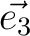). These values should be in radians. Example: MVEC 4 0.000000 0.000000 1.570796 0.000000 1.570796 1.570796 0.425741 0.418747 MAVE Specifies the number of directions of the applied magnetic field for the computation of the powder molar magnetization. The program will read two numbers: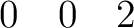and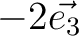.- number ofpoints in the interval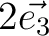. (Default 12)- number ofpoints in the interval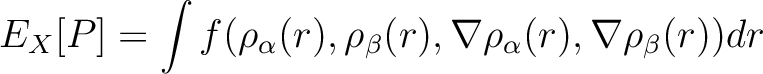. (Default 24) The number of directions over which the actual averaging will take place is roughly the product ofand. TEXP This keyword allows computation of the magnetic susceptibilityat experimental points. On the line below the keyword, the number of experimental points NT is defined, and on the next NT lines the program reads the experimental temperature (in K) and the experimental magnetic susceptibility (in cm3Kmol-1 ). TEXP and TINT keywords are mutually exclusive. The magnetic susceptibility routine will also print the standard deviation from the experiment. HEXP This keyword allows computation of the molar magnetization Mmol (H) at experimental points. On the line below the keyword,the number of experimental points NH is defined, and on the next NH lines the program reads the experimental field intensity (Tesla) and the experimental magnetization (in). HEXP and HINT are mutually exclusive. The magnetization routine will print the standard deviation from the experiment. ZJPR This keyword specifies the value (in cm-1) of a phenomenological parameter of a mean molecular field acting on the spin of the complex (the average intermolecular exchange constant). It is used in the calculation of all magnetic properties (not for spin Hamiltonians) (Default is 0.0) PRLV This keyword controls the print level. 2 - normal. (Default) 3 or larger (debug) POLY The program SINGLE_ANISO will prepare an input file (binary) for the future POLY_ANISO program. The default is not to create it. CRYS This keyword will enable computation of all 27 Crystal-Field parameters acting on the ground atomic multiplet of a lanthanide. On the next line the program will read the chemical symbol of the lanthanide. By default the program will not compute the parameters of the Crystal-Field. QUAX This keyword controls the quantization axis for the computation of the Crystal-Field parameters acting on the ground atomic multiplet of a lanthanide. On the next line, the program will read one of the three values: 1, 2 or 3. 1 - quantization axis is the main magnetic axis Zm of the ground pseudospin multiplet, whose size is specified within the MLTP keyword. (Default) 2 - quantization axis is the main magnetic axis Zm of the entire atomic multiplet |J,MJ>. 3 - the direction of the quantization axis is given by the user: on the next line the program will read three real numbers: the projections (px, py, pz) of the specified direction on the initial Cartesian axes. Note that px2 + py2 + pz2 = 1.

### 8.45.3.2 An input example

&SINGLE_ANISO
TITLe
magnetic  properties  for  Co  complex
TYPE
2
MVEC
3
0.000000 0.000000
1.570796 0.000000
1.570796 1.570796
MLTP
3
4  4  2
ZJPR
-0.2
ENCU
250  400
HINT
0.0  20.0  100  0.01
TINT
0.0  330.0  331  0.01
MAVE
16  18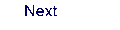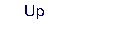Next: 8.46 SlapAf Up: 8. Programs Previous: 8.44 seward